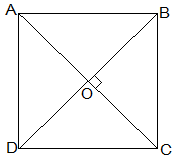Guru

# ABCD is a rhombus. Show that diagonal AC bisects ∠A as well as ∠C and diagonal BD bisects ∠B as well as ∠D. Q.7

• 0

Please give me the best way for solving the problem of class 9th ncert math of Quadrilaterals chapter of math of class 9th of exercise 8.1of question no 7  what is the tricky way for solving this question ABCD is a rhombus. Show that diagonal AC bisects ∠A as well as ∠C and diagonal BD bisects ∠B as well as ∠D.

Share

1.Given that,

ABCD is a rhombus.

AC and BD are its diagonals.

Proof,

AD = CD (Sides of a rhombus)

∠DAC = ∠DCA (Angles opposite of equal sides of a triangle are equal.)

also, AB || CD

⇒∠DAC = ∠BCA (Alternate interior angles)

⇒∠DCA = ∠BCA

, AC bisects ∠C.

Similarly,

We can prove that diagonal AC bisects ∠A.

Following the same method,

We can prove that the diagonal BD bisects ∠B and ∠D.

• 0